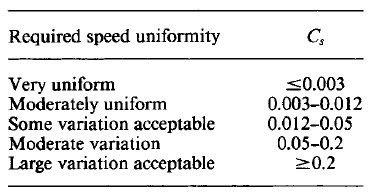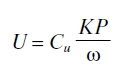Improve math skills of your kids - Learn step-by-step arithmetic from Math games

Math: Unknown - Step-by-step math calculation game for iOS.

Math: Unknown is much more than a math game. It is a step-by-step math calculation game which will teach users how to calculate in the correct order rather than just asking only the final calculated results.

The app consists of four basic arithmetic operations which are addition, subtraction, multiplication and division. In order to get started, users who are new to arithmetic can learn from animated calculation guides showing step-by-step procedures of solving each type of operation. It is also helpful for experienced users as a quick reference.

Generally, addition and subtraction may be difficult for users who just start learning math especially when questions require carrying or borrowing (also called regrouping). The app helps users to visualize the process of carrying and borrowing in the way it will be done on paper. Once users understand how these operations work, they are ready to learn multiplication and division.

For most students, division is considered as the most difficult arithmetic operation to solve. It is a common area of struggle since it requires prior knowledge of both multiplication and subtraction. To help users understand division, the app uses long division to teach all calculation procedures. Relevant multiplication table will be shown beside the question. Users will have to pick a number from the table which go into the dividend. Multiplication of selected number and divisor is automatically calculated, but the users have to do subtraction and drop down the next digit themselves. Learning whole calculation processes will make them master it in no time.

Math: Unknown is a helpful app for students who seriously want to improve arithmetic calculation skills.

Sizing the Flywheel

The energy-storage capacity of a flywheel is determined from its polar moment of inertia J and its maximum safe running speed.The necessary inertia depends on the cyclic torque variation and the allowable speed variation or, in the case of energystorage flywheels, the maximum energy requirements. The safe running speed depends on the geometry and material properties of the flywheel.

Flywheels store energy. Indeed, flywheels are used as energy reservoirs. Their principal use in machine design, however, is to smooth the variations in shaft speed that are caused by loads or power sources that vary in a cyclic fashion. By using its stored kinetic energy 0.5Jω2 to absorb the variations in torque during a machine cycle, a flywheel smooths the fluctuating speed of a machine and reduces undesirable transient loads. The effect of a flywheel is therefore fundamentally different from that of a regulator: A flywheel limits the speed variation over one cycle and has minimal effect on the average speed; a regulator uses negative feedback to maintain a selected average speed with only secondary effects on the speed during a cycle.

SIZING THE FLYWHEEL
A certain amount of fluctuation in shaft speed will not cause harmful torques or reduce the usefulness of a machine.The coefficient of speed fluctuation Cs is defined aswhere ω = rotational speed at the flywheel and ωavg = average of ωmax and ωmin. Ranges for Cs for several categories of speed variation are given in the following table. Assume that the system is stiff (the speeds of all shafts are proportional), that the external torque input or load is constant, and that ωavg is close to the constant speed at which the energy from the average torque balances the external energy (this is usually a good assumption for values of Cs up to about 0.2). The energy equation U = 0.5J(ω2max − ω2min) and the definition of Cs combine to give the equation for the required mass moment of inertiaThis inertia includes the flywheel inertia and the inertia of all rotating parts, referred to the flywheel speed by multiplying by the square of the ratio of the shaft speeds.If the torque-angle curve for a machine cycle is available from experimental data or a dynamic analysis, U is determined from the areas between the curve and the average-torque line. If the external torque input or load is not constant, it can be combined with the torque-angle curve for the machine. If the loading torque and the driving torque are not synchronized or have an unknown phase difference, a worstcase combination should be used. The areas under the curve can be determined using a planimeter or by graphic or numerical integration. Unless Cs is accurately known and the curve is from a worst case or is highly repeatable, precision in integrating is not warranted.

The torque-angle relationship for an engine depends on the fuel, gas pressures, reciprocating masses, speed, and engine geometry. The large variation that is possible between different engine designs shows that dynamic measurement or kinematic analysis is necessary to determine the torque fluctuation. It is often necessary, however, to come up with a rough estimate for preliminary design purposes or for checking the reasonableness of calculated values. For these purposes, the energy variation for an internal-combustion engine can be estimated bywhere K = 33000 lb.ft.rpm/hp [2π J⋅rad/(W⋅s)]